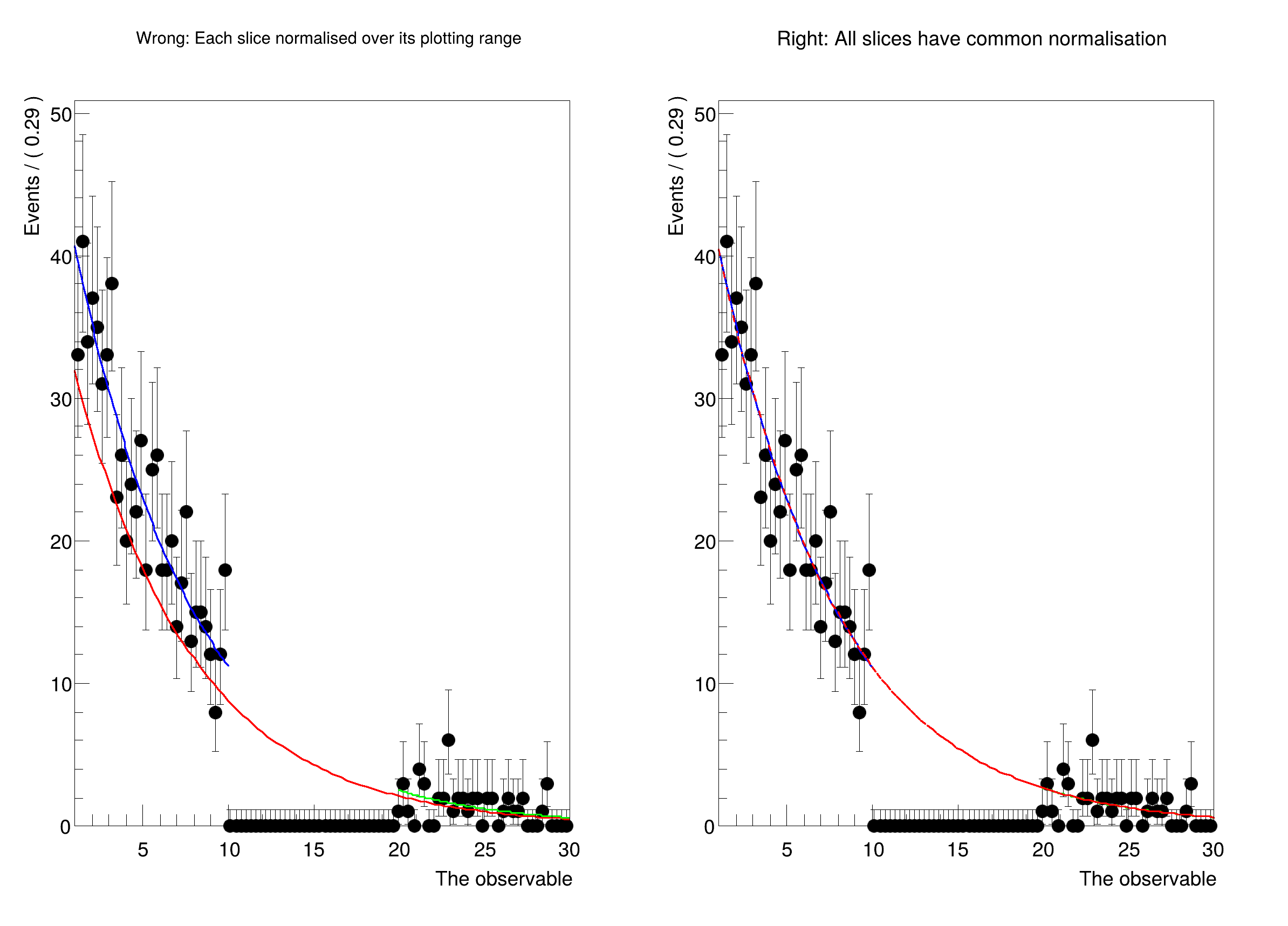ROOT   Reference Guide
Searching...
No Matches
rf212_plottingInRanges_blinding.py File Reference

## Namespaces

namespace  rf212_plottingInRanges_blinding

## Detailed DescriptionPlot a PDF in disjunct ranges, and get normalisation right.

Usually, when comparing a fit to data, one should first plot the data, and then the PDF. In this case, the PDF is automatically normalised to match the number of data events in the plot. However, when plotting only a sub-range, when e.g. a signal region has to be blinded, one has to exclude the blinded region from the computation of the normalisation.

In this tutorial, we show how to explicitly choose the normalisation when plotting using NormRange().import ROOT
# Make a fit model
x = ROOT.RooRealVar("x", "The observable", 1, 30)
tau = ROOT.RooRealVar("tau", "The exponent", -0.1337, -10.0, -0.1)
expo = ROOT.RooExponential("expo", "A falling exponential function", x, tau)
# Define the sidebands (e.g. background regions)
x.setRange("full", 1, 30)
x.setRange("left", 1, 10)
x.setRange("right", 20, 30)
# Generate toy data, and cut out the blinded region.
data = expo.generate(x, 1000)
blindedData = data.reduce(CutRange="left,right")
# Kick tau a bit, and run an unbinned fit where the blinded data are missing.
# ----------------------------------------------------------------------------------------------------------
# The fit should be done only in the unblinded regions, otherwise it would try
# to make the model adapt to the empty bins in the blinded region.
tau.setVal(-2.0)
expo.fitTo(blindedData, Range="left,right", PrintLevel=-1)
# Clear the "fitrange" attribute of the PDF. Otherwise, the fitrange would be
# automatically taken as the NormRange() for plotting. We want to avoid this,
# because the point of this tutorial is to show what can go wrong when the
# NormRange() is not specified.
expo.removeStringAttribute("fitrange")
# Here we will plot the results
canvas = ROOT.TCanvas("canvas", "canvas", 800, 600)
canvas.Divide(2, 1)
# Wrong:
# ----------------------------------------------------------------------------------------------------------
# Plotting each slice on its own normalises the PDF over its plotting range. For the full curve, that means
# that the blinded region where data is missing is included in the normalisation calculation. The PDF therefore
# comes out too low, and doesn't match up with the slices in the side bands, which are normalised to "their" data.
print("Now plotting with unique normalisation for each slice.\n")
canvas.cd(1)
plotFrame = x.frame(Title="Wrong: Each slice normalised over its plotting range")
# Plot only the blinded data, and then plot the PDF over the full range as well as both sidebands
blindedData.plotOn(plotFrame)
expo.plotOn(plotFrame, LineColor="r", Range="full")
expo.plotOn(plotFrame, LineColor="b", Range="left")
expo.plotOn(plotFrame, LineColor="g", Range="right")
plotFrame.Draw()
# Right:
# ----------------------------------------------------------------------------------------------------------
# Make the same plot, but normalise each piece with respect to the regions "left" AND "right". This requires setting
# a "NormRange", which tells RooFit over which range the PDF has to be integrated to normalise.
# This means that the normalisation of the blue and green curves is slightly different from the left plot,
# because they get a common scale factor.
print("\n\nNow plotting with correct norm ranges:\n")
canvas.cd(2)
plotFrameWithNormRange = x.frame(Title="Right: All slices have common normalisation")
# Plot only the blinded data, and then plot the PDF over the full range as well as both sidebands
blindedData.plotOn(plotFrameWithNormRange)
expo.plotOn(plotFrameWithNormRange, LineColor="b", Range="left", NormRange="left,right")
expo.plotOn(plotFrameWithNormRange, LineColor="g", Range="right", NormRange="left,right")
expo.plotOn(plotFrameWithNormRange, LineColor="r", Range="full", NormRange="left,right", LineStyle=10)
plotFrameWithNormRange.Draw()
canvas.Draw()
canvas.SaveAs("rf212_plottingInRanges_blinding.png")
[#1] INFO:Eval -- RooRealVar::setRange(x) new range named 'full' created with bounds [1,30]
[#1] INFO:Eval -- RooRealVar::setRange(x) new range named 'left' created with bounds [1,10]
[#1] INFO:Eval -- RooRealVar::setRange(x) new range named 'right' created with bounds [20,30]
[#1] INFO:Eval -- RooRealVar::setRange(x) new range named 'fit_nll_expo_expoData_left' created with bounds [1,10]
[#1] INFO:Eval -- RooRealVar::setRange(x) new range named 'fit_nll_expo_expoData_right' created with bounds [20,30]
[#1] INFO:Fitting -- RooAbsOptTestStatistic::ctor(nll_expo_expoData) constructing test statistic for sub-range named left,right
[#1] INFO:Minimization -- RooAbsMinimizerFcn::setOptimizeConst: activating const optimization
[#1] INFO:Minimization -- RooAbsMinimizerFcn::setOptimizeConst: deactivating const optimization
[#1] INFO:Plotting -- RooAbsPdf::plotOn(expo) only plotting range 'full', curve is normalized to data in given range
[#1] INFO:Plotting -- RooAbsPdf::plotOn(expo) only plotting range 'left', curve is normalized to data in given range
[#1] INFO:Plotting -- RooAbsPdf::plotOn(expo) only plotting range 'right', curve is normalized to data in given range
[#1] INFO:Plotting -- RooAbsPdf::plotOn(expo) only plotting range 'left'
[#1] INFO:Plotting -- RooAbsPdf::plotOn(expo) p.d.f. curve is normalized using explicit choice of ranges 'left,right'
[#1] INFO:Plotting -- RooAbsPdf::plotOn(expo) only plotting range 'right'
[#1] INFO:Plotting -- RooAbsPdf::plotOn(expo) p.d.f. curve is normalized using explicit choice of ranges 'left,right'
[#1] INFO:Plotting -- RooAbsPdf::plotOn(expo) only plotting range 'full'
[#1] INFO:Plotting -- RooAbsPdf::plotOn(expo) p.d.f. curve is normalized using explicit choice of ranges 'left,right'
Now plotting with unique normalisation for each slice.
Now plotting with correct norm ranges:
Date
June 2021

Definition in file rf212_plottingInRanges_blinding.py.